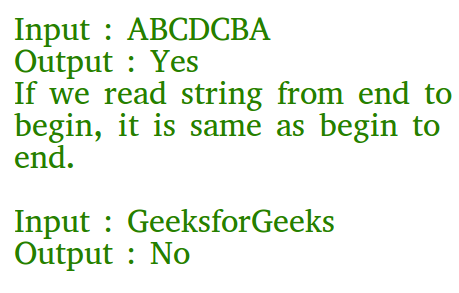# C Program to Check if a Given String is Palindrome

Given a string, write a c function to check if it is palindrome or not.
A string is said to be palindrome if reverse of the string is same as string. For example, “abba” is palindrome, but “abbc” is not palindrome.## Recommended: Please solve it on “PRACTICE ” first, before moving on to the solution.

Algorithm:
isPalindrome(str)
1) Find length of str. Let length be n.
2) Initialize low and high indexes as 0 and n-1 respectively.
3) Do following while low index ‘l’ is smaller than high index ‘h’.
…..a) If str[l] is not same as str[h], then return false.
…..b) Increment l and decrement h, i.e., do l++ and h–.
4) If we reach here, it means we didn’t find a mis

Following is C implementation to check if a given string is palindrome or not.

 `#include ` `#include ` ` `  `// A function to check if a string str is palindrome ` `void` `isPalindrome(``char` `str[]) ` `{ ` `    ``// Start from leftmost and rightmost corners of str ` `    ``int` `l = 0; ` `    ``int` `h = ``strlen``(str) - 1; ` ` `  `    ``// Keep comparing characters while they are same ` `    ``while` `(h > l) ` `    ``{ ` `        ``if` `(str[l++] != str[h--]) ` `        ``{ ` `            ``printf``(``"%s is Not Palindrome"``, str); ` `            ``return``; ` `        ``} ` `    ``} ` `    ``printf``(``"%s is palindrome"``, str); ` `} ` ` `  `// Driver program to test above function ` `int` `main() ` `{ ` `    ``isPalindrome(``"abba"``); ` `    ``isPalindrome(``"abbccbba"``); ` `    ``isPalindrome(``"geeks"``); ` `    ``return` `0; ` `}`

Output:

```abba is palindrome
abbccbba is palindrome
geeks is Not Palindrome```

Recursive function to check if a string is palindrome